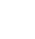# Adding a search function to a UserForm

We will need to know where the data we want to search is held, in this example it’s in the tab named ‘OrderFormData’ in columns B and C. Because INSTR is case sensitive, the way to be sure we don’t miss any text capitalisation changes in the data, we convert each to ‘virtual’ upper case. The listbox is setup with 4 columns, and we must manually hide column 1 which must also be the bound column, using the column width settings in the userform design.

We setup the form using this structure of a searchbox named ‘search’ a command button named ‘CommandButton1’ and a listbox named ‘Listbox1’.The following code example clears the form controls named F1 to F19 and the label named ‘rownum’ becomes blank. The code searches down column B of the OrderFormData sheet and populates the list (adds item) to the list from the value in column A when it matches. It also puts the values from columns B,C & E alongside, giving 4 columns of data. It then runs down column C and adds further matches to the list in the same structure.

Private Sub CommandButton1_Click()
On Error Resume Next
X = UCase(Search)

Rownum = “”

For n = 1 To 19
Me.Controls(“F” & n) = “”
Next n

ListBox1.Clear
With Sheets(“OrderFormData”)
lastrow = .Cells(.Rows.Count, “A”).End(xlUp).Row
End With

‘Search down column B
For n = 1 To lastrow

Y = Worksheets(“OrderFormData”).Range(“B” & n)
Z = UCase(Worksheets(“OrderFormData”).Range(“B” & n))
If InStr(1, Z, X) > 0 Then

‘Populate listbox
With ListBox1
.ColumnCount = 4
.List(ListBox1.ListCount – 1, 1) = Worksheets(“OrderFormData”).Range(“B” & n)
.List(ListBox1.ListCount – 1, 2) = Worksheets(“OrderFormData”).Range(“C” & n)
.List(ListBox1.ListCount – 1, 3) = Worksheets(“OrderFormData”).Range(“E” & n)
End With

End If

Next n

‘Search down column C
For n = 1 To lastrow

Y = Worksheets(“OrderFormData”).Range(“B” & n)
Z = UCase(Worksheets(“OrderFormData”).Range(“C” & n))
If InStr(1, Z, X) > 0 Then

‘Populate listbox
With ListBox1
.ColumnCount = 4
.List(ListBox1.ListCount – 1, 1) = Worksheets(“OrderFormData”).Range(“B” & n)
.List(ListBox1.ListCount – 1, 2) = Worksheets(“OrderFormData”).Range(“C” & n)
.List(ListBox1.ListCount – 1, 3) = Worksheets(“OrderFormData”).Range(“E” & n)
End With

End If

Next n

End Sub

## Upon ‘Double-clicking’ the listbox

The double clicking will populate the form with the record relating to the listbox value (ie the ref from column A). This action can be assigned to a double click, a single click or a command button if you wish and other events can be triggered by this code.

Private Sub ListBox1_DblClick(ByVal Cancel As MSForms.ReturnBoolean)
On Error Resume Next
d = ListBox1.Value

p = WorksheetFunction.Match(d, Worksheets(“OrderFormData”).Range(“A:A”), 0)

Rownum = p

For n = 1 To 19
Me.Controls(“F” & n) = Worksheets(“OrderFormData”).Cells(p, n)
Next n

End Sub